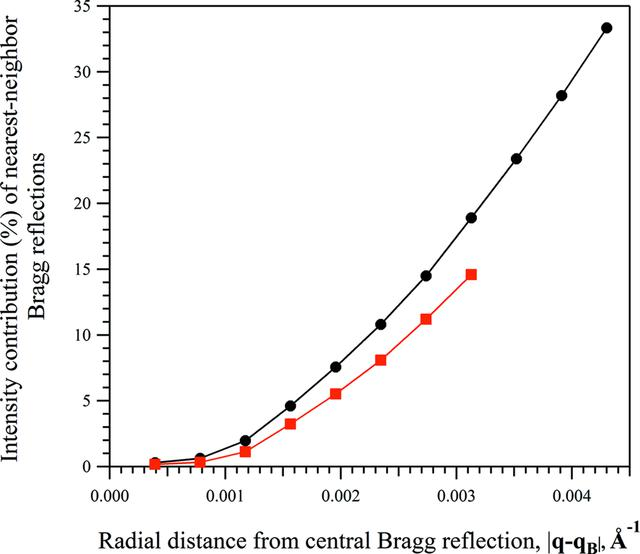disable zoom     view article Figure 4 The percentage contribution of nearest-neighbour Bragg reflections to the total modelled intensity distribution of the (980) Bragg reflection [|FWPF(980)| = 555.6, |FC(980)| = 551.1]. The percentage contribution was calculated as the percentage of the intensity distribution modelled by the surrounding Bragg reflections [the second term of equation (10)] relative to the total intensity modelled at radial distances from the Bragg position of the (980) reflection. The analysis was performed on two merged diffraction patterns generated from a collection of 2000 nanoscale crystals of the test protein with different mean unit-cell dimensions along the Y direction and with a mean of 10 unit cells along the X direction, 〈NX〉 = 10: (black, circles) 〈NY〉 = 30, (red, squares) 〈NY〉 = 60.

IUCrJ
Volume 3| Part 2| March 2016| Pages 127-138
ISSN: 2052-2525
Follow IUCrJ